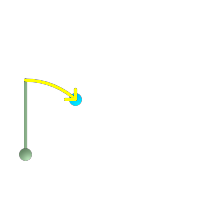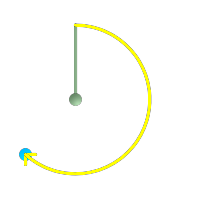## Name

ST_Azimuth — Returns the north-based azimuth as the angle in radians measured clockwise from the vertical on pointA to pointB.

## Synopsis

`float ST_Azimuth(`geometry pointA, geometry pointB`)`;

`float ST_Azimuth(`geography pointA, geography pointB`)`;

## Description

Returns the azimuth in radians of the segment defined by the given point geometries, or NULL if the two points are coincident. The azimuth is angle is referenced from north, and is positive clockwise: North = 0; Northeast = π/4; East = π/2; Southeast = 3π/4; South = π; Southwest 5π/4; West = 3π/2; Northwest = 7π/4.

For the geography type, the forward azimuth is solved as part of the inverse geodesic problem.

The azimuth is mathematical concept defined as the angle between a reference plane and a point, with angular units in radians. Units can be converted to degrees using a built-in PostgreSQL function degrees(), as shown in the example.

Availability: 1.1.0

Enhanced: 2.0.0 support for geography was introduced.

Enhanced: 2.2.0 measurement on spheroid performed with GeographicLib for improved accuracy and robustness. Requires Proj >= 4.9.0 to take advantage of the new feature.

Azimuth is especially useful in conjunction with ST_Translate for shifting an object along its perpendicular axis. See upgis_lineshift Plpgsqlfunctions PostGIS wiki section for example of this.

## Examples

Geometry Azimuth in degrees

```SELECT degrees(ST_Azimuth(ST_Point(25, 45), ST_Point(75, 100))) AS degA_B,
degrees(ST_Azimuth(ST_Point(75, 100), ST_Point(25, 45))) AS degB_A;

dega_b       |     degb_a
------------------+------------------
42.2736890060937 | 222.273689006094
```Green: the start Point(25,45) with its vertical. Yellow: degA_B as the path to travel (azimuth).Green: the start Point(75,100) with its vertical. Yellow: degB_A as the path to travel (azimuth).